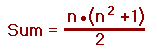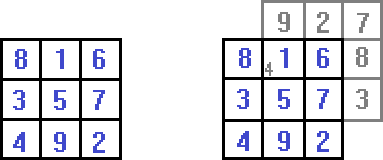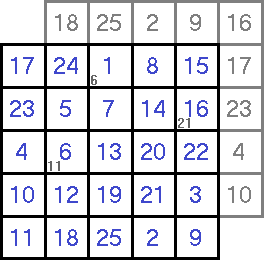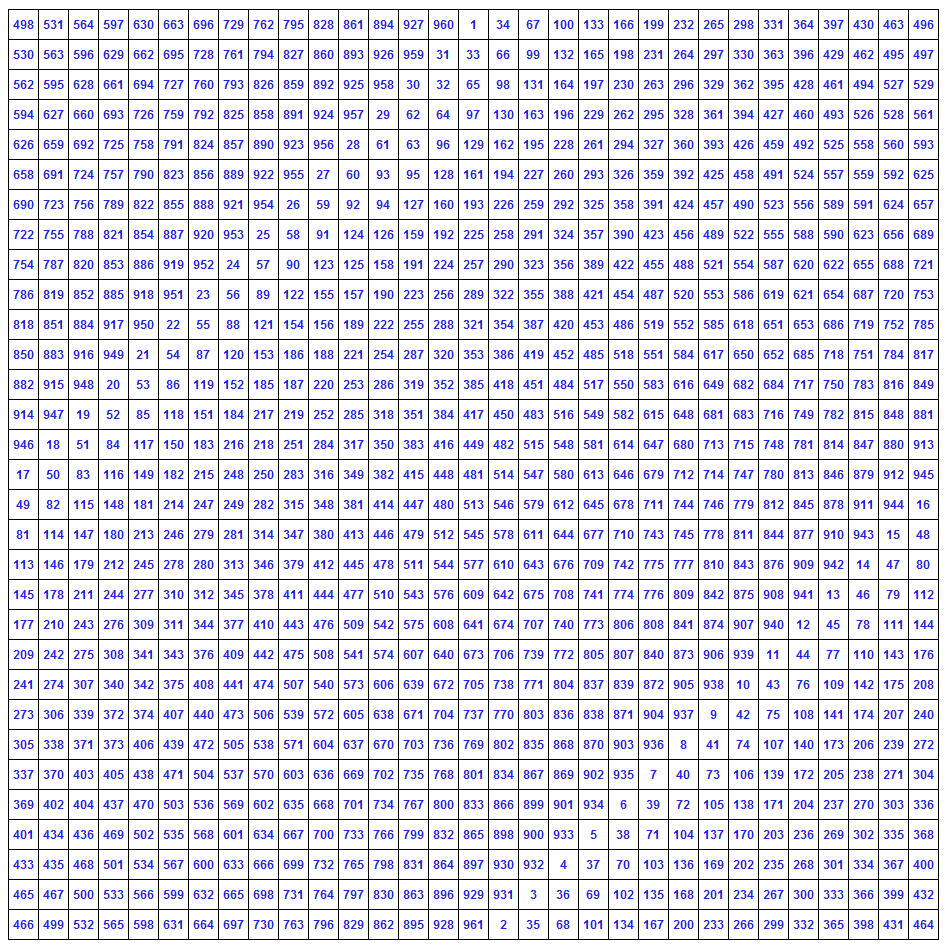Links to Other Magic Square Pages:
Even Magic Squares
Singly Even Magic Squares

# Odd Magic Squares

The typical (or normal) magic square consists of consecutive integers (starting with 1 and ending with n²) placed into 'n' rows by 'n' columns so that all rows, all columns and both diagonals sum to the same total.

A 1 by 1 magic square contains just the number 1 and is so simplistic, it is not worth discussing.
It is impossible to construct a 2 by 2 magic square (n = 2) and so the first magic square worth discussing occurs when n = 3.

#### Odd Magic Squares

A 3 by 3 magic square is an odd magic square (n=3, 5, 7, 9, 11, etc), one of the three types of magic square.

The other two types are:
doubly even (multiple of 4 where n=4, 8, 12, 16, 20, etc.)
singly even (even but not a multiple of 4 where n=6, 10, 14, 18, 22, etc.)

On this page, we will discuss how to construct odd magic squares, beginning with the 3 row by 3 column magic square.
To determine the sum of any normal magic square we use the formula:So, for the 3 by 3 magic square, each row, each column and both diagonals would sum to
3 • (3² + 1) ÷ 2 = 15

The most popular and most well-known method for creating odd magic squares was first published by Simon de la Loubère (1642-1729) and it is this method being illustrated here.The left diagram shows a completed 3 by 3 magic square and the right diagram shows how it was created.

1) The number '1' goes in the middle of the top row.

2) All numbers are then placed one column to the right and one row up from the previous number.

Looking at the diagram on the right, going up and to the right of number '1', we see that the '2' will end up outside of the square. (note the gray '2') In a sense, the '2' is in the correct column but too high to be in a row and so we have another rule for placing the numbers:

3) Whenever the next number placement is above the top row, stay in that column and place the number in the bottom row.

Going up and to the right of '2' we have the number '3' outside of the square (note the gray '3') and so we need another new rule.

4) Whenever the next number placement is outside of the rightmost column, stay in that row and place the number in the leftmost column.

Going up and to the right of number '3', the location where the '4' should go (note the gray '4') has already been filled in by the number '1'. This is the first time that this has occurred and you should be careful where you place the next number. Remember at this point, the '5' is not yet filled in and many people make the mistake of putting the next number where the '5' is. The rule for this is:

5) When encountering a filled-in square, place the next number directly below the previous number.

There is no problem with placing the '5' and the '6' but the '7' ends up in the topmost and rightmost position in the diagram (note the gray '7'). When this occurs, the rule is quite similar to rule 5:

6) When the next number position is outside both a row and a column, place the number directly beneath the previous number.

The placement of the '8' and the '9' are based upon rules four and three respectively and now the magic square has been completed.

If you feel you need to practice a bit more, here is a 5 by 5 magic square with the 'hint' numbers included.To show that Simon de la Loubère's method works for any size odd magic square, here is one where n = 31 and each row, column and both diagonals sum to 14,911.